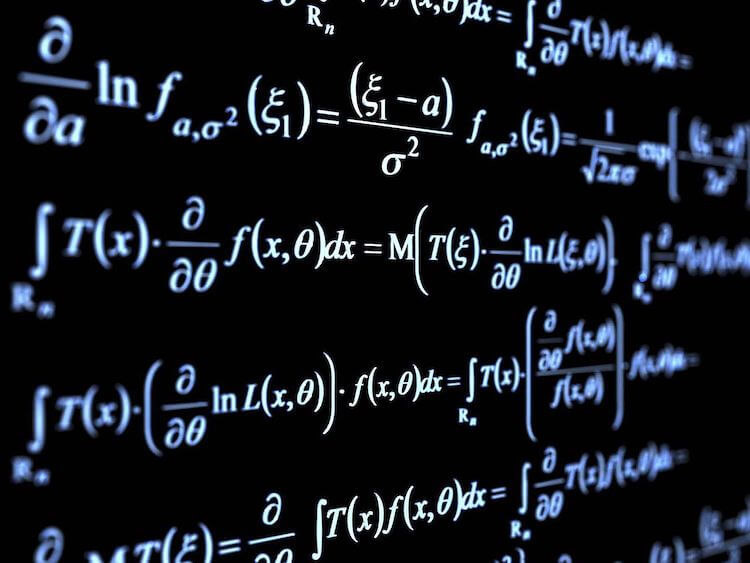Not clear about what Differentiation is in mathematics? Attend the live classes of Cuemath. It is an online platform that offers several live and recorded lectures for the Math program. Teachers here are well-experienced and have all the necessary knowledge about the basics of Math. Here in this article, we have discussed the basic concepts of differentiation

## What is the Meaning of Differentiation in Maths?

In Mathematics Differentiation can be defined as the rate of change of one quantity in relation to the other one. In calculus, Differentiation holds an important place apart from integration. It is the method of finding the derivative of a function. In calculus, Differentiation is applied to calculate the function per unit change in the variable that is independent.

When y = f(x) is the function of x then, the rate of change of ‘y’ with respect to the per-unit change in ‘x’ is given by dy / dx or f’(x).

If f(x), the function goes through any change of ‘h’ near any point of ‘x’ then the derivative of the function is given by:

Notations: When the function is expressed as y=f(x), then the derivative can be expressed by the given notations:

1. The Euler’s notation D(y) or D[f(x)]
2. The Lagrange’s notation F’(x)
3. The Leibniz’s notation dy/dx

## Applications of Differentiation in Real Life

1. It helps to find the value of acceleration i.e. rate of change in the velocity in relation to the given time.
2. To note variations in the temperature.
3. It helps to find the tangent and is normal to a curve.
4. The derivative function can be used to calculate the highest and lowest point of a curve in the graph.
5. To calculate the amount of profit or loss earned by the business using a graph.

## What are the Formulas to Calculate Differentiation?

Let us consider f(x), the function and f’(x), the derivative of the function. The vital formulas to calculate Differentiation is a follow:

1. When f(x) = tan (x), then f'(x) = sec2x
2. When f(x) = sin (x), then f'(x) = cos x
3. When f(x) = cos (x), then f'(x) = -sin x
4. When f(x) = exex, then f'(x) = exex
5. When f(x) = ln(x), then f'(x) = 1/x
6. When f(x) = xnxn, where n is any fraction or integer, then f'(x) = nxn−1nxn−1
7. When f(x) = k, where k is a constant, then f'(x) = 0

## What Are the Rules of Differentiation?

The important rules of Differentiation that should be followed are given below:

1. Sum and Difference Rule
2. Product Rule
3. Quotient Rule
4. Chain Rule

Sum or Difference Rule: According to this rule, when the given function f(x) is the sum or difference of the two functions u(x) and v(x) then the derivative of the function f’(x) is the sum or difference of two given individual functions u’(x) and v’(x).

when f(x) = u(x) ± v(x)

then, f'(x) = u'(x) ± v'(x)

Product Rule: According to this rule, when the given function f(x) is the product of the two functions u(x) and v(x) then the derivative of the function f’(x) is,

when f(x) = u(x) × v(x)

then, f′(x) = u′(x) × v(x) + u(x) × v′(x)

Quotient Rule: According to this rule, when the given function f(x) is the division of the two functions u(x) and v(x) then the derivative of the function f’(x) is,

when, f(x)=u(x) / v(x)

then f(x’) = u’(x) × v(x) – u(x) × v’(x) / (v(x)2

Chain Rule: According to this rule, when the given function y = f(x) = g(u) and when u = h(x), then,

dydx = dydu × dudx

### The Bottom Line

There are so many real-life situations, especially in professional life when you may need to apply the differentiation principles and formulas. For instance, calculating the profit and loss in business using graphs, measuring the temperature variation, calculating the speed or distance covered such as miles per hour, kilometer per hour, etc, and a lot more.

So, even if you have not studied it with a keen interest in your student life, learning it now can be a great help to grow and advance your career.

Differentiation is a complicated concept. Through this article, we have discussed its meaning, differentiation formula, its real-life application, and rules of differentiation and now with clear basic concepts of differentiation, you can solve difficult mathematical problems related to your work and avoid a significant amount of workplace stress.